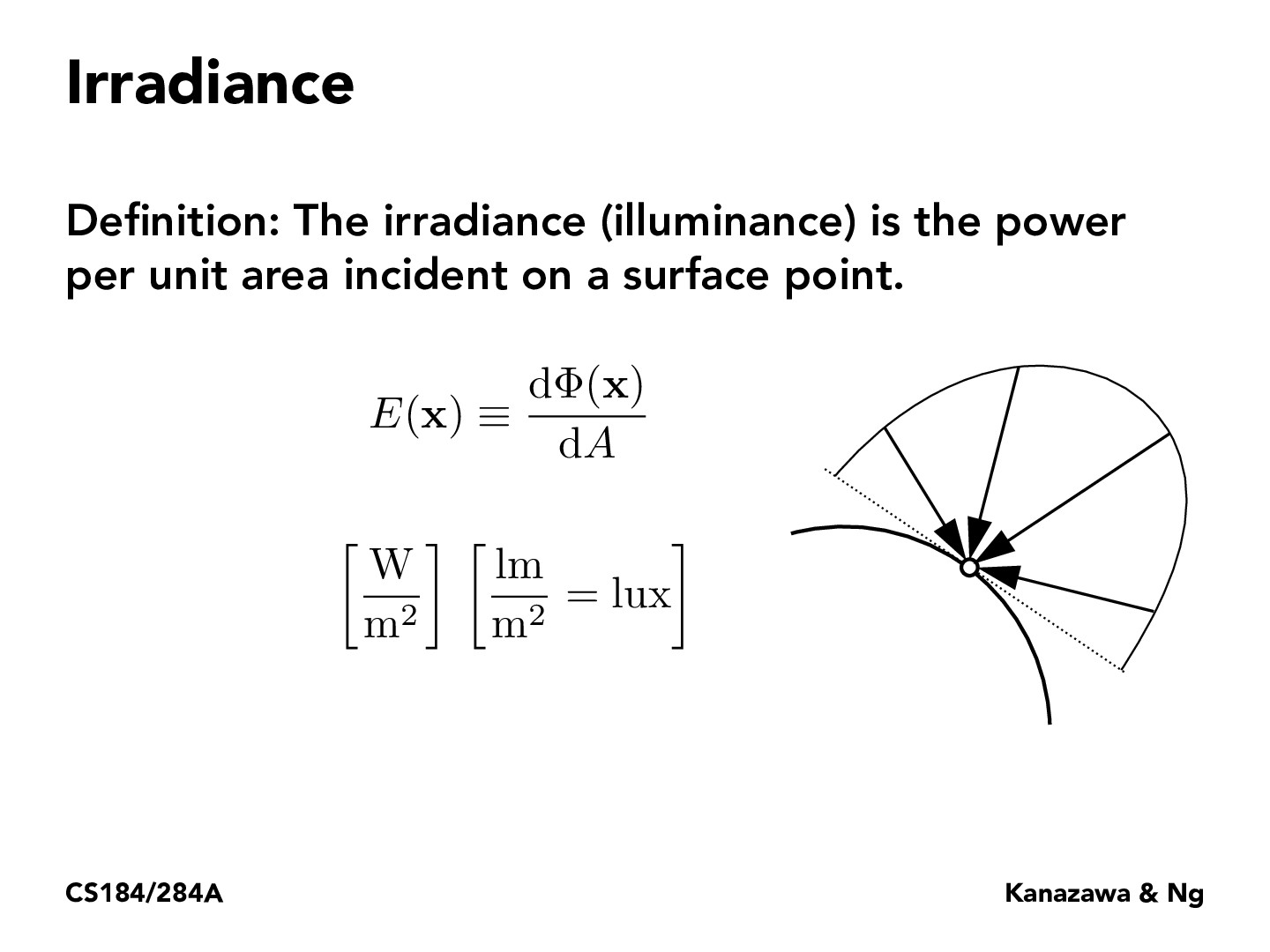Lecture 11: Radiometry & Photometry (25)PongsatornChan

How is this different from the intensity in the sense that it is energy per area instead of per solid angle? Or is it intensity on the receiving end instead of intensity from the source of light?YIYANGCAI

I believe that there is some connection between irradiance and radiant intensity. Because I think irradiance is the reverse process (in some ways) of the radiant intensity. Image a sphere like mirror which reflect all light from a light source back.Leon-Shao

I'm thinking about the angle of the light and whether we can put it into the equation where we just take the perpendicular direction of the light to the surface point?pixelled

@Leon-Shao Yes, we can just take the perpendicular direction of the light to the surface, and this is exactly the Lambert's Law, which says that the amount of light energy arriving at a surface is proportional to the cosine of the angle between the light direction and the surface normal. Hence, irradiance varies according to the cosine of the incidence of the illumination.ZizhengTai

@PongsatornChan, isn't it that irradiance is talking about how much light we are receiving at a point, while intensity is how much light we are emitting from a point? To get the irradiance for a certain point, we would need to consider the intensity of all the light sources that can reach this point.

You must be enrolled in the course to comment